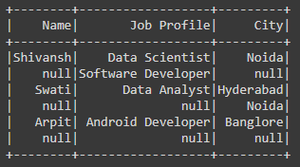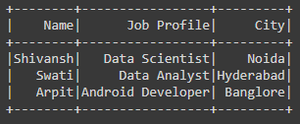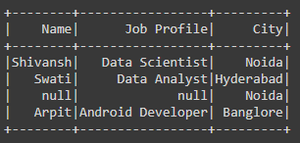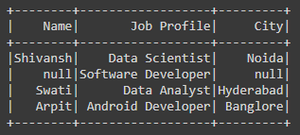# Filter PySpark DataFrame Columns with None or Null Values

• Last Updated : 09 May, 2021

Many times while working on PySpark SQL dataframe, the dataframes contains many NULL/None values in columns, in many of the cases before performing any of the operations of the dataframe firstly we have to handle the NULL/None values in order to get the desired result or output, we have to filter those NULL values from the dataframe.

In this article are going to learn how to filter the PySpark dataframe column with NULL/None values.

For filtering the NULL/None values we have the function in PySpark API know as a filter() and with this function, we are using isNotNull() function.

Syntax:

• df.filter(condition) : This function returns the new dataframe with the values which satisfies the given condition.
• df.column_name.isNotNull() : This function is used to filter the rows that are not NULL/None in the dataframe column.

Example 1: Filtering PySpark dataframe column with None value

In the below code we have created the Spark Session, and then we have created the Dataframe which contains some None values in every column. Now, we have filtered the None values present in the Name column using filter() in which we have passed the condition df.Name.isNotNull() to filter the None values of Name column.

## Python

 `# importing necessary libraries``from` `pyspark.sql ``import` `SparkSession`` ` `# function to create SparkSession``def` `create_session():``    ``spk ``=` `SparkSession.builder \``        ``.master(``"local"``) \``        ``.appName(``"Filter_values.com"``) \``        ``.getOrCreate()``    ``return` `spk`` ` `# function to create dataframe``def` `create_df(spark, data, schema):``    ``df1 ``=` `spark.createDataFrame(data, schema)``    ``return` `df1`` ` ` ` `if` `__name__ ``=``=` `"__main__"``:`` ` `    ``# calling function to create SparkSession``    ``spark ``=` `create_session()`` ` `    ``input_data ``=` `[(``"Shivansh"``, ``"Data Scientist"``, ``"Noida"``),``                  ``(``None``, ``"Software Developer"``, ``None``),``                  ``(``"Swati"``, ``"Data Analyst"``, ``"Hyderabad"``),``                  ``(``None``, ``None``, ``"Noida"``),``                  ``(``"Arpit"``, ``"Android Developer"``, ``"Banglore"``),``                  ``(``None``, ``None``, ``None``)]``     ` `    ``schema ``=` `[``"Name"``, ``"Job Profile"``, ``"City"``]`` ` `    ``# calling function to create dataframe``    ``df ``=` `create_df(spark, input_data, schema)`` ` `    ``# filtering the columns with None values``    ``df ``=` `df.``filter``(df.Name.isNotNull())`` ` `    ``# visulizing the dataframe``    ``df.show()`

Output:Original DataframeDataframe after filtering NULL/None values

Example 2: Filtering PySpark dataframe column with NULL/None values using filter() function

In the below code we have created the Spark Session, and then we have created the Dataframe which contains some None values in every column. Now, we have filtered the None values present in the City column using filter() in which we have passed the condition in English language form i.e, “City is Not Null” This is the condition to filter the None values of the City column.

Note: The condition must be in double-quotes.

## Python

 `# importing necessary libraries``from` `pyspark.sql ``import` `SparkSession`` ` `# function to create new SparkSession``def` `create_session():``    ``spk ``=` `SparkSession.builder \``        ``.master(``"local"``) \``        ``.appName(``"Filter_values.com"``) \``        ``.getOrCreate()``    ``return` `spk`` ` ` ` `def` `create_df(spark, data, schema):``    ``df1 ``=` `spark.createDataFrame(data, schema)``    ``return` `df1`` ` ` ` `if` `__name__ ``=``=` `"__main__"``:`` ` `    ``# calling function to create SparkSession``    ``spark ``=` `create_session()`` ` `    ``input_data ``=` `[(``"Shivansh"``, ``"Data Scientist"``, ``"Noida"``),``                  ``(``None``, ``"Software Developer"``, ``None``),``                  ``(``"Swati"``, ``"Data Analyst"``, ``"Hyderabad"``),``                  ``(``None``, ``None``, ``"Noida"``),``                  ``(``"Arpit"``, ``"Android Developer"``, ``"Banglore"``),``                  ``(``None``, ``None``, ``None``)]``     ` `    ``schema ``=` `[``"Name"``, ``"Job Profile"``, ``"City"``]`` ` `    ``# calling function to create dataframe``    ``df ``=` `create_df(spark, input_data, schema)`` ` `    ``# filtering the columns with None values``    ``df ``=` `df.``filter``(``"City is Not NULL"``)`` ` `    ``# visulizing the dataframe``    ``df.show()`

Output:Original DataframeAfter filtering NULL/None values from the city column

Example 3: Filter columns with None values using filter() when column name has space

In the below code, we have created the Spark Session, and then we have created the Dataframe which contains some None values in every column. We have filtered the None values present in the ‘Job Profile’ column using filter() function in which we have passed the condition df[“Job Profile”].isNotNull() to filter the None values of the Job Profile column.

Note: For accessing the column name which has space between the words, is accessed by using square brackets [] means with reference to the dataframe we have to give the name using square brackets.

## Python

 `# importing necessary libraries``from` `pyspark.sql ``import` `SparkSession`` ` `# function to create SparkSession``def` `create_session():``    ``spk ``=` `SparkSession.builder \``        ``.master(``"local"``) \``        ``.appName(``"Filter_values.com"``) \``        ``.getOrCreate()``    ``return` `spk`` ` ` ` `def` `create_df(spark, data, schema):``    ``df1 ``=` `spark.createDataFrame(data, schema)``    ``return` `df1`` ` ` ` `if` `__name__ ``=``=` `"__main__"``:`` ` `    ``# calling function to create SparkSession``    ``spark ``=` `create_session()`` ` `    ``input_data ``=` `[(``"Shivansh"``, ``"Data Scientist"``, ``"Noida"``),``                  ``(``None``, ``"Software Developer"``, ``None``),``                  ``(``"Swati"``, ``"Data Analyst"``, ``"Hyderabad"``),``                  ``(``None``, ``None``, ``"Noida"``),``                  ``(``"Arpit"``, ``"Android Developer"``, ``"Banglore"``),``                  ``(``None``, ``None``, ``None``)]``     ` `    ``schema ``=` `[``"Name"``, ``"Job Profile"``, ``"City"``]`` ` `    ``# calling function to create dataframe``    ``df ``=` `create_df(spark, input_data, schema)`` ` `    ``# filtering the Job Profile with None values``    ``df ``=` `df.``filter``(df[``"Job Profile"``].isNotNull())`` ` `    ``# visulizing the dataframe``    ``df.show()`

Output:Original DataframeAfter filtering NULL/None values from the Job Profile column

My Personal Notes arrow_drop_up